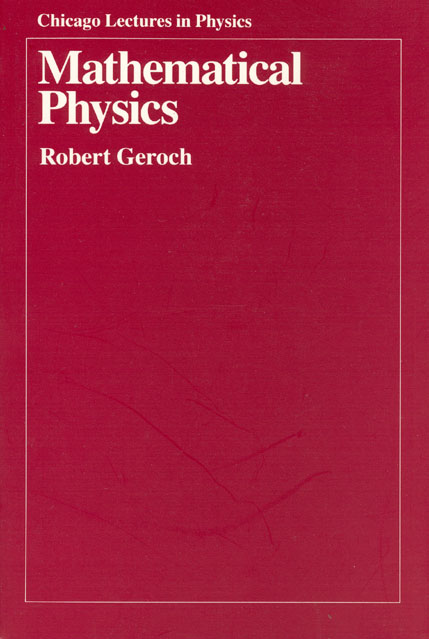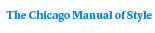# Mathematical Physics

Robert Geroch358 pages | 6 x 9 | © 1984
Paper \$41.00 ISBN: 9780226288628 Published September 1985
E-book \$10.00 to \$41.00 About E-books ISBN: 9780226223063 Published August 2015
Mathematical Physics is an introduction to such basic mathematical structures as groups, vector spaces, topological spaces, measure spaces, and Hilbert space. Geroch uses category theory to emphasize both the interrelationships among different structures and the unity of mathematics. Perhaps the most valuable feature of the book is the illuminating intuitive discussion of the "whys" of proofs and of axioms and definitions. This book, based on Geroch’s University of Chicago course, will be especially helpful to those working in theoretical physics, including such areas as relativity, particle physics, and astrophysics.
Table of Contents• Contents
Contents
1. Introduction
2. Categories
3. The Category of Groups
4. Subgroups
5. Normal Subgroups
6. Homomorphisms
7. Direct Products and Sums of Groups
8. Relations
9. The Category of Vector Spaces
10. Subspaces
11. Linear Mappings; Direct Products and Sums
12. From Real to Complex Vector Spaces and Back
13. Duals
14. Multilinear Mappings; Tensor Products
15. Example: Minkowski Vector Space
16. Example: The Lorentz Group
17. Functors
18. The Category of Associative Algebras
19. The Category of Lie Algebras
20. Example: The Algebra of Observables
21. Example: Fock Vector Space
22. Representations: General Theory
23. Representations on Vector Spaces
24. The Algebraic Categories: Summary
25. Subsets and Mappings
26. Topological Spaces
27. Continuous Mappings
28. The Category of Topological Spaces
29. Nets
30. Compactness
31. The Compact-Open Topology
32. Connectedness
33. Example: Dynamical Systems
34. Homotopy
35. Homology
36. Homology: Relation to Homotopy
37. The Homology Functors
38. Uniform Spaces
39. The Completion of a Uniform Space
40. Topological Groups
41. Topological Vector Spaces
42. Categories: Summary
43. Measure Spaces
44. Constructing Measure Spaces
45. Measurable Functions
46. Integrals
47. Distributions
48. Hilbert Spaces
49. Bounded Operators
50. The Spectrum of a Bounded Operator
51. The Spectral Theorem: Finite-dimensional Case
52. Continuous Functions of a Hermitian Operator
53. Other Functions of a Hermitian Operator
54. The Spectral Theorem
55. Operators (Not Necessarily Bounded)